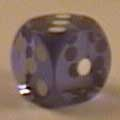<< Home

# Homework

Homeworks will be released weekly. It is mandatory to typeset your solutions using LaTeX. There is a template linked below.Homework 0 (LaTeX) (Solutions)Homework 1 (LaTeX) (Solutions)Homework 2 (LaTeX) (Solutions)Homework 3 (LaTeX) (Solutions)Homework 4 (LaTeX) (Solutions)Homework 5 (LaTeX) (Solutions)Homework 6 (LaTeX) (Solutions))Midterm (LaTeX) (Solutions))Homework 8 (LaTeX) (Solutions)Homework 9 (LaTeX) (Solutions)Homework 10 (LaTeX)(Solutions)Homework 11 (LaTeX)(Solutions)Final (LaTeX)(Solutions)

# LaTeXTemplate for writing solutions in LaTeX: hw-skel.texBrown CS Latex pageLast Updated: Jan 2020